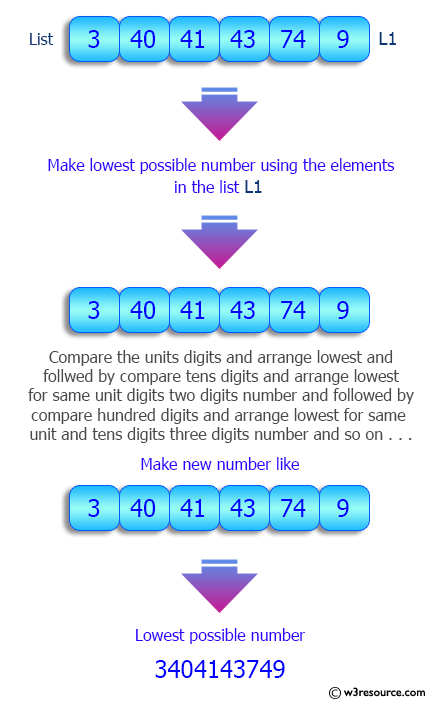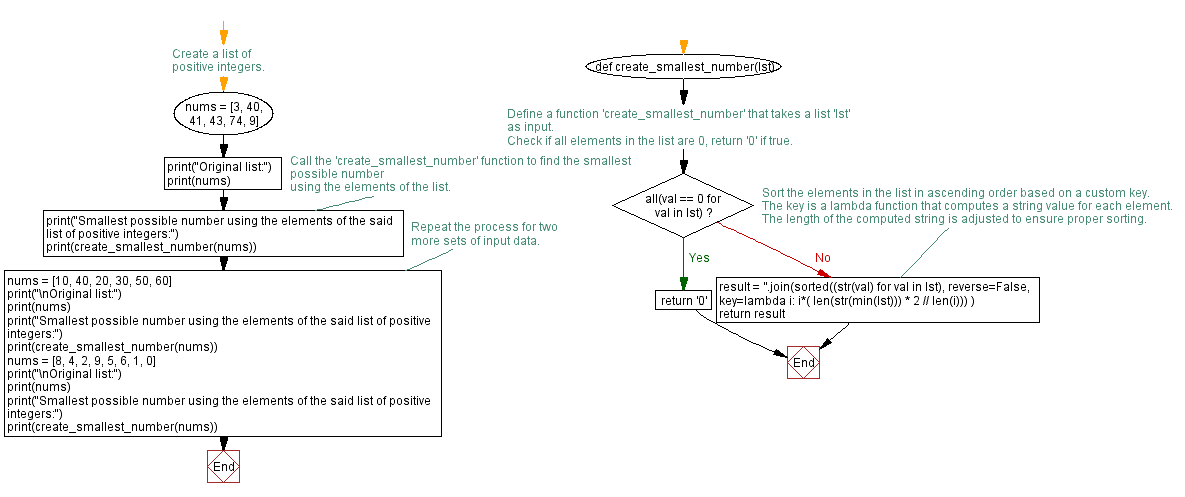﻿ Python: Create the smallest possible number using the elements of a given list of integers - w3resource# Python: Create the smallest possible number using the elements of a given list of integers

## Python List: Exercise - 180 with Solution

Write a Python program to create the smallest possible number using the elements of a given list of positive integers.

Sample Solution:

Python Code:

``````def create_largest_number(lst):
if all(val == 0 for val in lst):
return '0'
result = ''.join(sorted((str(val) for val in lst), reverse=False,
key=lambda i: i*( len(str(min(lst))) * 2 // len(i))))
return result

nums = [3, 40, 41, 43, 74, 9]
print("Original list:")
print(nums)
print("Smallest possible number using the elements of the said list of positive integers:")
print(create_largest_number(nums))

nums = [10, 40, 20, 30, 50, 60]
print("\nOriginal list:")
print(nums)
print("Smallest possible number using the elements of the said list of positive integers:")
print(create_largest_number(nums))
nums = [8, 4, 2, 9, 5, 6, 1, 0]
print("\nOriginal list:")
print(nums)
print("Smallest possible number using the elements of the said list of positive integers:")
print(create_largest_number(nums))
```
```

Sample Output:

```Original list:
[3, 40, 41, 43, 74, 9]
Smallest possible number using the elements of the said list of positive integers:
3404143749

Original list:
[10, 40, 20, 30, 50, 60]
Smallest possible number using the elements of the said list of positive integers:
102030405060

Original list:
[8, 4, 2, 9, 5, 6, 1, 0]
Smallest possible number using the elements of the said list of positive integers:
01245689
```

Pictorial Presentation:Flowchart:## Visualize Python code execution:

The following tool visualize what the computer is doing step-by-step as it executes the said program:

Python Code Editor:

Have another way to solve this solution? Contribute your code (and comments) through Disqus.

What is the difficulty level of this exercise?

Test your Python skills with w3resource's quiz

﻿

## Python: Tips of the Day

Floor Division:

When we speak of division we normally mean (/) float division operator, this will give a precise result in float format with decimals.

For a rounded integer result there is (//) floor division operator in Python. Floor division will only give integer results that are round numbers.

```print(1000 // 300)
print(1000 / 300)```

Output:

```3
3.3333333333333335```